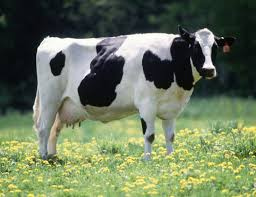# Dataset 1: Cow diets• credit: commons.wikimedia

As written in the module. These data are from an experiment on cows. Cows were split into 6 groups, each given a different diet treatment. Here you will look at how these different treatments influenced the dry matter intake (DMI) of the cows.

You can find the data here: it is a .csv with a header.

Important! When you import the data it is important to make sure it is in the right format. Here you need Treatment to be a factor and Baseline to be numeric. See code below.

``````cowdata <- read.csv("https://www.math.ntnu.no/emner/ST2304/2020v/Week09/cowdata.csv", header=T)

# str() checks the data structure
str(cowdata)

# we can see that the variables are not the right format
# so we fix it
cowdata\$Treatment <- as.factor(cowdata\$Treatment)
cowdata\$Baseline <- as.numeric(cowdata\$Baseline)

# now check again
str(cowdata)
``````

The columns in the dataframe are:

• DMI = the dry matter intake during the experiment (grams)
• Baseline = the baseline dry matter intake before the experiment (grams)
• Treatment = the diet treatment group (1 to 6)

You want to find out how diet treatment influenced dry matter intake while controlling for the baseline intake of each cow.

1. What model will you use to answer this? (1 mark)

2. What type of variables do you have and which are response or explanatory? (3 marks)

We have given code to run a model for the data below. Think about what type of model is being run? It is good practice to consider if you would have chosen the same one.

``````# Model 1
model1 <- lm(DMI ~ Treatment+Baseline, data = cowdata)

coef(model1)

confint(model1)
``````

3. Interpret the output of the model. What does it tell you about the effect of diet on DMI? (5 marks)

Below is the code to make some graphs to check the model fit.

``````# Graph 1

residuals <- residuals(model1)
fitted <- fitted(model1)
plot(fitted, residuals)

qqnorm(residuals)
qqline(residuals)
``````

4. What are the assumptions of the model? (5 marks)

5. Are the assumptions met? Reference which plot you use to decide and why you make the choice. (6 marks)

6. What other plot might you also want for checking assumptions? (1 mark)

Here is code for another model on the same data.

``````# Model 2
model2 <- lm(DMI ~ Treatment*Baseline, data = cowdata)

coef(model2)

confint(model2)
``````

7. How is this model different to the first one? (1 mark)

8. Given the new model, does this change your interpretation of the effect of diet on DMI? Why? (4 marks)

9. Which model do you prefer, why? (4 marks)

Show me the answers